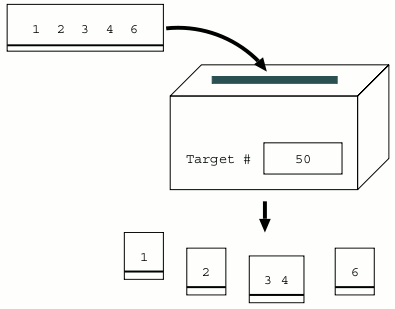Shredding Company

Time Limit : 8 sec, Memory Limit : 131072 KB

Problem F: Shredding Company

You have just been put in charge of developing a new shredder for the Shredding Company. Although a ``normal'' shredder would just shred sheets of paper into little pieces so that the contents would become unreadable, this new shredder needs to have the following unusual basic characteristics.

• The shredder takes as input a target number and a sheet of paper with a number written on it.
• It shreds (or cuts) the sheet into pieces each of which has one or more digits on it.
• The sum of the numbers written on each piece is the closest possible number to the target number, without going over it.

For example, suppose that the target number is 50, and the sheet of paper has the number 12346. The shredder would cut the sheet into four pieces, where one piece has 1, another has 2, the third has 34, and the fourth has 6. This is because their sum 43 (= 1 + 2 + 34 + 6) is closest to the target number 50 of all possible combinations without going over 50. For example, a combination where the pieces are 1, 23, 4, and 6 is not valid, because the sum of this combination 34 (= 1 + 23 + 4 + 6) is less than the above combination's 43. The combination of 12, 34, and 6 is not valid either, because the sum 52 (= 12+34+6) is greater than the target number of 50.Figure 1. Shredding a sheet of paper having the number 12346 when the target number is 50

There are also three special rules:

• If the target number is the same as the number on the sheet of paper, then the paper is not cut. For example, if the target number is 100 and the number on the sheet of paper is also 100, then the paper is not cut.
• If it is not possible to make any combination whose sum is less than or equal to the target number, then error is printed on a display. For example, if the target number is 1 and the number on the sheet of paper is 123, it is not possible to make any valid combination, as the combination with the smallest possible sum is 1, 2, 3. The sum for this combination is 6, which is greater than the target number, and thus error is printed.
• If there is more than one possible combination where the sum is closest to the target number without going over it, then rejected is printed on a display. For example, if the target number is 15, and the number on the sheet of paper is 111, then there are two possible combinations with the highest possible sum of 12: (a) 1 and 11 and (b) 11 and 1; thus rejected is printed.

In order to develop such a shredder, you have decided to first make a simple program that would simulate the above characteristics and rules. Given two numbers, where the first is the target number and the second is the number on the sheet of paper to be shredded, you need to figure out how the shredder should ``cut up'' the second number.

Input

The input consists of several test cases, each on one line, as follows:

```t1 num1
t2 num2
...
tn numn
0 0
```

Each test case consists of the following two positive integers, which are separated by one space: (1) the first integer (ti above) is the target number; (2) the second integer (numi above) is the number that is on the paper to be shredded.

Neither integers may have a 0 as the first digit, e.g., 123 is allowed but 0123 is not. You may assume that both integers are at most 6 digits in length. A line consisting of two zeros signals the end of the input.

Output

For each test case in the input, the corresponding output takes one of the following three types:

• sum part1 part2 ...
• rejected
• error

In the first type, partj and sum have the following meaning:

• Each partj is a number on one piece of shredded paper. The order of partj corresponds to the order of the original digits on the sheet of paper.
• sum is the sum of the numbers after being shredded, i.e., sum = part1 + part2 + ... .

Each number should be separated by one space.

The message "error" is printed if it is not possible to make any combination, and "rejected" if there is more than one possible combination.

No extra characters including spaces are allowed at the beginning of each line, nor at the end of each line.

Sample Input

```50 12346
376 144139
927438 927438
18 3312
9 3142
25 1299
111 33333
103 862150
6 1104
0 0
```

Output for the Sample Input

```43 1 2 34 6
283 144 139
927438 927438
18 3 3 12
error
21 1 2 9 9
rejected
103 86 2 15 0
rejected
```

Source: ACM International Collegiate Programming Contest , Asia Regional Kanazawa, Kanazawa, Japan, 2002-11-04
http://www.kitnet.jp/icpc/